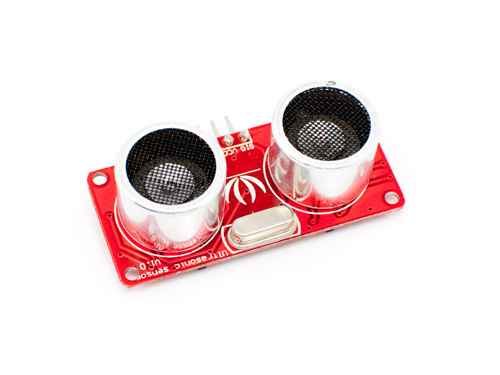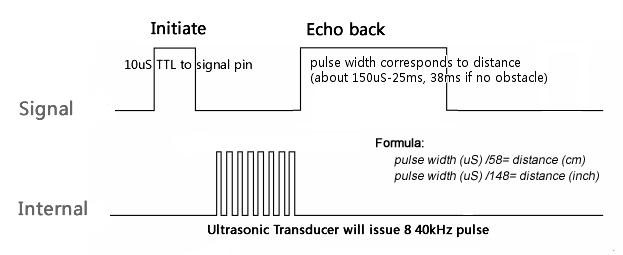# Arduino and ultra sonic range measurement module or how to measure the pulse time with a hardware timer and an interruptSEN136B5B is a Ultra Sonic range measurement from SeedStudio. It can measure the distance by sending a 40k Hz ultra sound impulse via the transmitter  and calculating time needed for echo to reach receiver. Detecting range: 3cm-4m. More information about device.

In the description of module is mentioned that it is compatible with Arduino library, but I decided to write program without using PulseIn command.As you can see from picture above reading information from ultra sonic module requires measurement of time. So once again I have used a hardware Atmega 16 bit timer/counter.

```void setup()   {

Serial.begin(9600);
Serial.print(0x0C,BYTE); // clear screen

TIMSK1=0x01; // enabled global and timer overflow interrupt;
TCCR1A = 0x00; // normal operation page 148 (mode0);
TCCR1B=0; //stop
}
```

I have used the serial OLED display GLO-16 to show distance, so there is serial port configuration. I hope to review this display later at the separate blog post.

The configuration of timer is standard.

```void loop() {

state=0;
overflow_counter=0;

pinMode(sense_pin, OUTPUT);
digitalWrite(sense_pin,HIGH);
delayMicroseconds(10); // 10 us impulse to start measurement

pinMode(sense_pin, INPUT);
digitalWrite(21,LOW);

attachInterrupt(interrupt_pin, interrupt, RISING);
x=0;

//waiting for interrupt

delay(50);

```

At the beginning of the loop a positive 10 µs duration impulse is generated at pin 21(there is the SIG pin of ultra sonic module connected)  to start measurement. Then the interrupt for rising edge is enabled. Not all Arduino pins can trigger external interrupt.

```#define interrupt_pin 21 //SIG
#define interrupt_number 2
```

```
void interrupt()
{
if (!x) { // rising edge happened

TCCR1B=2; //start timer with /8 prescaler
attachInterrupt(interrupt_number, interrupt, FALLING);

}

else {  //falling edge happened
TCCR1B=0; //stop timer
count=TCNT1;
TCNT1=0x000;
state=1;

}

x=~x;
}

```

After external interrupt is triggered by rising edge, timer is started and interrupt for falling edge is enabled.

With the /8 prescaler timer/counter is clocked at 2 MHz rate. A counter increases by 1 every 0.5 µs. That is 0.5 * 2^16 = 32,7 ms until overflow. It is enough if object is in 4 meter range, but it’s not if object if out of range and module generates 38 ms impulse, so the program lets timer to overflow one time.

If the falling edge is detected, timer is stopped and content of his register is saved.

```
void manage_overflow () {

if (overflow_counter>1 ) {

detachInterrupt(interrupt_number);
TCCR1B=0; //stop
state=2;
}

}

ISR(TIMER1_OVF_vect) {

overflow_counter++;
manage_overflow();

}

```

If falling interrupt doesn’t occur since 32,7 ms since rising interrupt, one timer overflow is allowed. If ~ 60 ms is not enough timer is stopped and error is displayed.

Let’s back to end of loop:

```
switch(state) {

case 1:
{

time=count*0.5; // µs

if ( overflow_counter!=0) {
show_out_off_range();
}

else  show_distance(time/58);

break;
}

case 2:
{
show_error();
break;
}

default :
show_error();

}

delay(250);
};

```

state = 0 – no measurement are performed, module is not connected.

state =1 – it means that measurement was successful. Time is converted to distance, but if timer’s overflow occurred once then it’s likely that module generated 38 ms signal – no obstacle.

state = 2 – error, since time between rising and falling edges is more than ~60ms.

All program:

```//Ultra Sonic range measurement module SEN136B5B
// with GLO-216 2x16 Multifont Serial OLED
//electronicsblog.net
unsigned char x=0;
double count =0;
double time=0;
int state=0;
int overflow_counter=0;

#define interrupt_pin 21 //SIG
#define interrupt_number 2

void show_error(void) {

Serial.print(0x0C,BYTE); // clear screen

Serial.print("Error!");
Serial.print(0x01,BYTE); //home cursor
Serial.print(0x0A,BYTE); //move down one row
Serial.print("Check wiring!");
}

void show_out_off_range(void){

Serial.print(0x0C,BYTE); // clear screen

Serial.print("No obstacle!");
}

void show_distance(double distance)

{

Serial.print(0x01,BYTE); //home cursor
Serial.print(0x03,BYTE); // normal font
Serial.print(0x02,BYTE);// increase font
Serial.print(0x02,BYTE);// increase font
Serial.print(0x02,BYTE);// increase font

Serial.print(distance);

if (distance<10) {
Serial.print("   ");

Serial.print(0x08,BYTE); // back space
Serial.print(0x08,BYTE); // back space
Serial.print(0x08,BYTE); // back space
}

if (distance<100) {
Serial.print("  ");

Serial.print(0x08,BYTE); // back space
Serial.print(0x08,BYTE); // back space
}

Serial.print(0x03,BYTE);  // normal font
Serial.print(0x0A,BYTE); //move down one row
Serial.print(" cm");
if (distance<100) {
Serial.print("   ");
}
if (distance<10) {
Serial.print(" ");
}

}

void interrupt()
{
if (!x) { // rising edge happened

TCCR1B=2; //start timer with /8 prescaler
attachInterrupt(interrupt_number, interrupt, FALLING);

}

else {  //falling edge happened

TCCR1B=0; //stop timer
count=TCNT1;
TCNT1=0x000;
state=1;

}

x=~x;
}

void manage_overflow () {

if (overflow_counter>1 ) {

detachInterrupt(interrupt_number);
TCCR1B=0; //stop
state=2;
}

}

ISR(TIMER1_OVF_vect) {

overflow_counter++;
manage_overflow();

}

void setup()   {

Serial.begin(9600);
Serial.print(0x0C,BYTE); // clear screen

TIMSK1=0x01; // enabled global and timer overflow interrupt;
TCCR1A = 0x00; // normal operation page 148 (mode0);
TCCR1B=0; //stop
}

void loop() {

state=0;
overflow_counter=0;

pinMode(interrupt_pin, OUTPUT);
digitalWrite(interrupt_pin,HIGH);
delayMicroseconds(10); // 10 us impulse to start measurement

pinMode(interrupt_pin, INPUT);
digitalWrite(21,LOW);

attachInterrupt(interrupt_number, interrupt, RISING);
x=0;

//waiting for interrupt

delay(50);

switch(state) {

case 1:
{

time=count*0.5; // µs

if ( overflow_counter!=0) {
show_out_off_range();
}

else  show_distance(time/58);

break;
}

case 2:
{
show_error();
break;
}

default :
show_error();

}

delay(250);
};

```

Video demonstration is below: